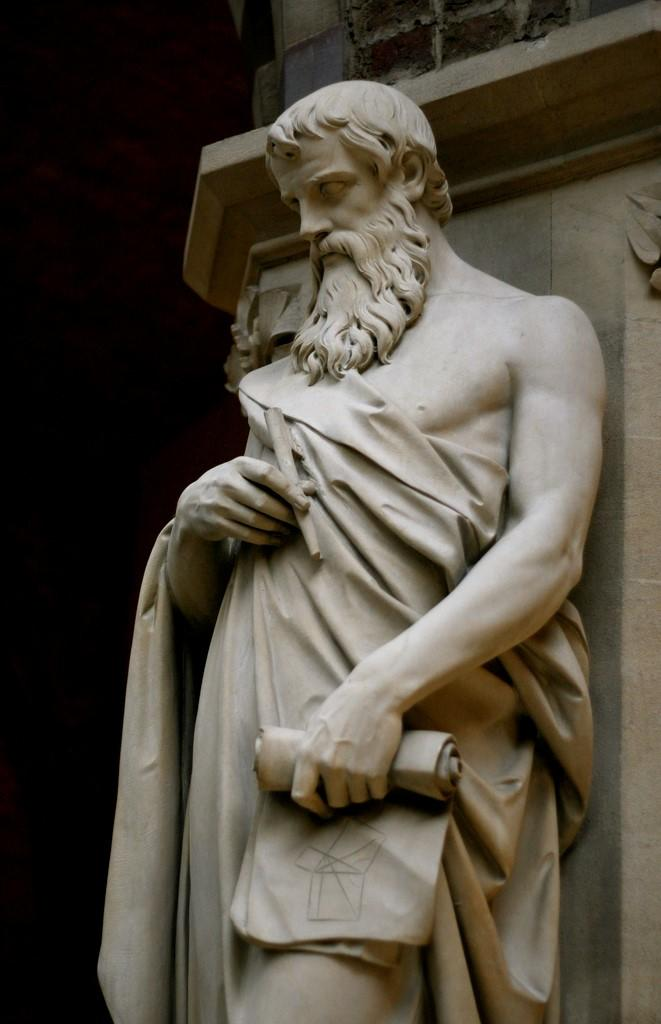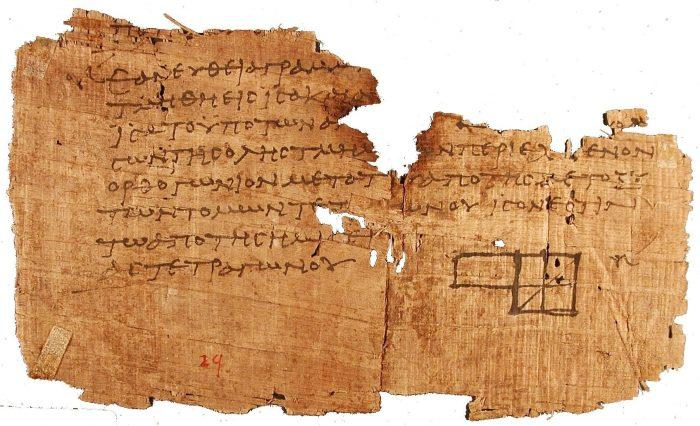Over the centuries, the history of mathematics has witnessed the rise of many greats: Pythagoras, Thales, Newton, Archimedes. Here, we will be discussing the infamous Euclid. A great mathematician from antiquity, Euclid managed to revolutionize all of the established body of knowledge of his era in one sole work: Elements. In this way, Euclid forms the basis of mathematics as we learn it today. Trigonometry, algebraic reasoning, equation, fraction, logarithm – all of these aspects of math are still marked by the mathematicians of antiquity. Euclid’s axiom, Euclidean division, Euclidean geometry, Euclid’s algorithm - keep reading to find out about the history of math throughout its various scientific discoveries.

## The Life of Euclid the Mathematician

Similar to his predecessors Pythagoras and Thales, the history of Euclid is not well documented. Only some written texts dating many years after Euclid’s death have been found, and it is only from these that we have managed to capture historical fragments of the career of the now famous mathematician, Euclid. There is no other mathematician from antiquity that is more celebrated Euclid. Born in Athens around 330 BCE, Euclid’s studies were formed in the beautiful Egyptian city of Alexandria, then under the reign of king Ptolemy I. There, Euclid frequented the notorious Museum of Alexandria, the hub of intellectual life in the city. Unlike his predecessors, Euclid did not create his own school for mathematics. However, the scientist certainly had many students and disciples that formed his own personal entourage, to whom he passed down all of his knowledge and wisdom. This entourage also took part in developing many of his experiments. One legend has it that Euclid had given an insignificant amount of change to one of his students while he had asked him what Euclid was getting out of his mathematical research. In other words, Euclid was not in search of money. Instead of big fortunes, the mathematician preferred to nourish his brain with mathematical formulas and numbers of all types.Euclidean thought inspired antiquity and contemporary philosophers alike Euclid is known most for his work entitled Elements that was written around 300 BCE. Both a success in his times and in today’s world, this work was the second most printed book, after the bible, at time of the invention of the printer of the 15th century. Elements, divided into thirteen books, is dedicated essentially to plane geometry and arithmetic. Triangles, parallel lines, circles – Euclid proved many theorems in his book (including the Pythagoras theorem), introduced notions of GCD (greatest common divisor) and introduced repeated, successive subtractions which are now known as Euclidean divisions. These could be seen as the predecessor to what Isaac Newton would later create: calculus! Euclid’s knowledge was based on knowledge that had long been acquired from the greatest mathematicians of antiquity. In this epoque, sciences were making their way around Greece and influenced a great number of budding scientists. Euclid and his contemporaries’ discoveries continued to inspire the sciences a long time after his death, estimated at around 265 BCE in Alexandria. For more famous mathematicians from antiquity, check out the great Archimedes!

## Euclid's Seminal Text Elements

Even though he wrote some other influential works, Elements is considered to be Euclid’s main work. A great scientific success, the mathematician catalogues in this work all of the proofs of geometric knowledge that were ever known until then. The first six books in Elements deal with the geometric plane. Here, we find information about triangles, parallel lines, the Pythagorean theorem, planes, the properties of a circle (in the presence of figures in a circle), the construction of the Pentagon and the proportions between its sizes. These first books are widely recognized as one of the first moments in which the basics of geometry are detailed with the characteristics of figures and their applications. This later formed the basis of the creation of analytic geometry by Rene Descartes! The three books that follow don’t deal with geometry but with arithmetic. In this section, Euclid discusses prime numbers, the construction of the GCD of two or more integers, numbers in geometric progression, and the construction of perfect numbers. This first section of chapters in Elements is also known for its introduction of a repeated, successive subtraction process now called Euclidean division.Fragments left over from the original publication of Elements The second book is dedicated to irrational quantities. It is comprised of the three last books, which are devoted to geometry in space. Here, we see the construction of objects like the sphere, regular solids, the pyramid, the cube, the octahedron, the dodecahedron, the icosahedron, etc. Other books have been grafted onto Elements during the course of its centuries long. These were written by new mathematicians that wanted to develop and add onto the chapters therein. All of the books of the body of work Elements form the basis of the curriculum of mathematics that are taught today. The geometric plane, geometry in space, arithmetic – these all form part of the mathematical courses given from primary school to higher education, which is the reason why Elements is considered the Bible for mathematics. The seminal work was long considered as the reference material for the mathematical world before being re-discussed centuries later. All of the information given by Elements can be seen as a photograph of the representation of the physical world in Euclid’s time. Find a math tutor online with Superprof.

## What is Euclidean Division?

In the section of coursework and curriculum dedicated to arithmetic, Euclidean division is certainly one of the mathematical skills that is still taught from antiquity. It is nothing more than the division that one learns in elementary school. Also called whole division, it is composed of an operation between two whole numbers called a dividend and a divisor, which gives the results called the quotient and the remainder. Making a Euclidean division of one number A (the dividend) by one number B (the divisor), permits us to try to find a whole quotient. That is to say, the whole integer found at the end of the division is called the remainder, which is the part of the dividend that we cannot divide further. To better understand, here’s an example: With the dividend of 25, we divide by 4 (the divisor). The quotient turns out to be 6 because 6 X 4 =24. What is left over is 1. The number 1, then, is the remainder. The common way to write this type of division is to place the dividend on the left side and the divisor on the right. The remainder is found under the dividend while the quotient is found under the divisor. To verify that you have finished the operation, make sure that the remainder cannot be divided further. It will be necessarily smaller than the divisor. It could be that the remainder is zero. In this case, we say that A is a multiple of B. Euclidean division is an integral part of our elementary courses, although this division can and does get more complicated with the addition of decimals, etc. Find Waterloo math courses with a tutor from Superprof.

## Euclid's Mathematical Axioms

In his work Elements Euclid wrote many axioms, which are mathematical propositions that are held as evident. It was at this moment in history that the mathematical world decided to designate name “axiom” to all mathematical rules that were at once elementary and logical. Euclid gave 5 in his work:

1. “A straight line segment can be drawn joining any two points.
2. “Any straight line segment can be extended indefinitely in a straight line.
3. “Given any straight line segment, a circle can be drawn having the segment as radius and one endpoint as center.
4. All right angles are congruent.

## What You Should Know About the Greatest Common Divisor

The Euclidean algorithm is also an important concept taught in mathematics courses and deals with the highest common factor. Also called the greatest common denominator, the GCD is the biggest common divisor between two integers. Just like Euclidean division, it is also considered to be a part of simple arithmetic. To find the GCD, it is necessary to make a list of all the divisors that two numbers possess. For example, take 10 and 26:

• 10: 1,2,5,10
• 26: 1,2,4,9,13

In this case, the greatest common divisor would be the number 2. In order to avoid having to make a list of all the possible divisors for each number, the Euclidean algorithm consists of making a series of Euclidean divisions. Applying this concept, it suffices to simply divide the biggest number by the smallest one and proceed to divide until the remainder is equal to 0. The Euclidean algorithm is explained in book seven of Elements. Euclid first presents his research in the form of a geometric problem. He then searches to find the unit of measure for two segments. To do this, he decides to subtract the smallest segment by the biggest and to continue until he finds the ideal measure. This method is now the base of all division and the cause of many headaches in primary school!

Need a Math teacher?5.00/5 - 2 review(s)Loading...